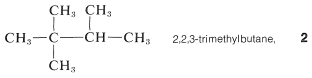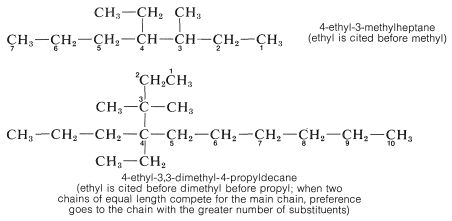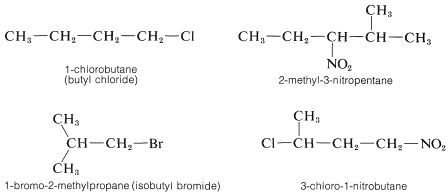# 3.2: Alkanes

$$\newcommand{\vecs}{\overset { \rightharpoonup} {\mathbf{#1}} }$$ $$\newcommand{\vecd}{\overset{-\!-\!\rightharpoonup}{\vphantom{a}\smash {#1}}}$$$$\newcommand{\id}{\mathrm{id}}$$ $$\newcommand{\Span}{\mathrm{span}}$$ $$\newcommand{\kernel}{\mathrm{null}\,}$$ $$\newcommand{\range}{\mathrm{range}\,}$$ $$\newcommand{\RealPart}{\mathrm{Re}}$$ $$\newcommand{\ImaginaryPart}{\mathrm{Im}}$$ $$\newcommand{\Argument}{\mathrm{Arg}}$$ $$\newcommand{\norm}{\| #1 \|}$$ $$\newcommand{\inner}{\langle #1, #2 \rangle}$$ $$\newcommand{\Span}{\mathrm{span}}$$ $$\newcommand{\id}{\mathrm{id}}$$ $$\newcommand{\Span}{\mathrm{span}}$$ $$\newcommand{\kernel}{\mathrm{null}\,}$$ $$\newcommand{\range}{\mathrm{range}\,}$$ $$\newcommand{\RealPart}{\mathrm{Re}}$$ $$\newcommand{\ImaginaryPart}{\mathrm{Im}}$$ $$\newcommand{\Argument}{\mathrm{Arg}}$$ $$\newcommand{\norm}{\| #1 \|}$$ $$\newcommand{\inner}{\langle #1, #2 \rangle}$$ $$\newcommand{\Span}{\mathrm{span}}$$$$\newcommand{\AA}{\unicode[.8,0]{x212B}}$$

The most definitive set of organic nomenclature rules currently in use were evolved through several international conferences and are known as the International Union of Pure and Applied Chemistry Rules (IUPAC rules). We first shall describe this system for naming the hydrocarbons known as alkanes - the so-called saturated paraffin hydrocarbons that have no double or triple bonds, or rings, and conform to the general formula $$C_nH_{2n+2}$$.

The alkanes are classified as "continuous chain" (that is, "unbranched") if all the carbon atoms in the chain are linked to no more than two other carbons; or "branched chain" if one or more carbon atoms are linked to more than two other carbons:The first four continuous-chain hydrocarbons have nonsystematic names:The higher members, beginning with pentane, are named systematically with a numerical prefix (pent-, hex-, hept-, etc., to denote the number of carbon atoms) and with the ending -ane to classify the compound as a paraffin hydrocarbon, as in Table 3-1. To specify a continuous-chain hydrocarbon, the prefix n- (for normal) sometimes is used. However, in the absence of any qualifying prefix, the hydrocarbon is considered to be "normal" or unbranched and we shall not use this prefix henceforth. You should memorize the names up to $$C_{10}H_{22}$$.The possibility of having branched-chain hydrocarbons that are structural isomers of the continuous-chain hydrocarbons begins with butane ($$n = 4$$). The IUPAC rules for the systematic naming of these hydrocarbons follow.

1. The longest continuous chain of carbon atoms is taken as the parent hydrocarbon and is the framework on which the various substituent groups are attached. Thus the hydrocarbon $$1$$ is a substituted pentane rather than a substituted butane because the longest chain has five carbons:2. The substituent groups attached to the main chain are named by replacing the ending -ane of the alkane by -yl. We then have the alkyl groups(or alkyl radicals, the simplest examples being the methyl ($$CH_3-$$) and ethyl ($$CH_3CH_3-$$) groups.

3. The parent hydrocarbon then is numbered starting from the end of the chain, and the substituent groups are assigned numbers corresponding to their positions on the chain. The direction of numbering is chosen to give the lowest numbers to the side-chain substituents.$$^2$$ Thus hydrocarbon $$1$$ is 2,3-dimethylpentane rather than 3,4-dimethylpentane. The prefix di- signifies that there are two identical substituents:The prefixes used to designate the number of substituents follow up to ten.Mono- is not used to designate a single substituent in systematic nomenclature, but may be used in conversation for emphasis.

4. Where there are two identical substituents at one position as in $$2$$, numbers are supplied for each, and the prefix, di-, tri-, and so on, is included to signify the number of groups of the same kind:5. Branched-chain substituent groups are given appropriate names by a simple extension of the system used for branched-chain hydrocarbons. The longest chain of the substituent is numbered starting with the carbon attached directly to the parent hydrocarbon chain. Parentheses are used to separate the numbering of the substituent and of the main hydrocarbon chain:Additional examples of alkyl substituents and their names are listed in Table 3-2. These are further classified according to whether they are primary, secondary, or tertiary. An alkyl group is described as primary if the carbon at the point of attachment is bonded to only one other carbon, as secondary if bonded to two other carbons, and tertiary if bonded to three other carbons. Thus if $$R$$ is any hydrocarbon radical, the different kinds of alkyl groups areConfusion can arise here because we often refer to specific carbons rather than whole alkyl groups as primary, secondary, and so on. In this context, a carbon is primary if it is bonded to one other carbon, secondary if bonded to two, tertiary if bonded to three, and quaternary if bonded to four. Thus, either carbon of ethane is primary, the $$C2$$ carbon in propane, $$CH_3CH_2CH_3$$, is secondary, and the $$C2$$ carbon of 2,2-dimethylpropane, $$(CH_3)_4C$$, is quaternary.

The situation with regard to naming alkyl substituents has been muddied considerably by the fact that the IUPAC rules allow use of trivial names for a few alkyl groups. Thus sec-butyl sometimes is used in place of 1-methylpropyl, and tert-butyl in place of 1,1-dimethylethyl These and other examples are included in parentheses in Table 3-2. Further odd-ball but less official customs are the prefix iso, which is reserved for substituents with two methyl groups at the end of an otherwise straight chain (e.g., isopropyl), and the prefix neo,which is used to denote three methyl groups at the end of a chain (e.g., neopentyl, which is more properly called 2,2-dimethylpropyl). Also in common use are the names isobutane and neopentane for the hydrocarbons 2-methyl-propane and 2,2-dimethylpropane, respectively There is no ambiguity involved in the use of iso and neo prefixes here, but the practice of using the name "isooctane" for 2,2,4-trimethylpentane is erroneous. Fortunately, use of these special names is declining.6. When there are two or more different substituents present, the question arises as to what order they should be cited in naming the compound. The system adopted by IUPAC and long practiced by Chemical Abstracts cites them in alphabetical order without regard for whether there is a multiplying prefix such as di- or tri-. Examples are given below.When a hydrocarbon is substituted with other than alkyl groups a new problem arises, which can be illustrated by $$CH_3CH_2Cl$$ This substance can be called either chloroethane or ethyl chloride, and both names are used in conversation and in print almost interchangeably. In the IUPAC system, halogens, nitro groups, and a few other monovalent groups are considered to be substituent groups on hydrocarbons and are named as haloalkanes, nitroalkanes, and so on.

The alphabetical order of precedence is preferred for substituents of different types when two or more are attached to a hydrocarbon chain because this makes indexing and using indexes more straightforward:The rest of this chapter is devoted to names of compounds we will not discuss for several chapters ahead and you may wish to stop at this point and return later as necessary. However, you should test your knowledge of alkane nomenclature by doing Exercises 3-1, 3-2, 3-3, 3-9, 3-10, and 3-11.

$$^2$$Confusion is possible when the numbering from each end is similar. The rule is that when the series of substituent locants are compared term by term, the "lowest" series has the lowest number at the first point of difference. The compoundis 2,3,5-trimethylhexane, not 2,4,5-trimethylhexane.